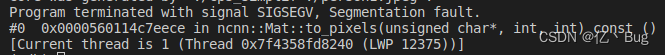# [Solved] C++ reason ncnn model error: Segmentation fault (core dumped)

The reasoning code is as follows:

``````#include "net.h"
#include <iostream>
#include <fstream>
#include <algorithm>
#include <opencv2/core/core.hpp>
#include <opencv2/highgui/highgui.hpp>
#include <opencv2/imgproc.hpp>
#include <stdio.h>
#include <vector>
#include <opencv2/opencv.hpp>

using namespace std;

void pretty_print(const ncnn::Mat& m)
{
for (int q=0; q<m.c; q++)
{
const float* ptr = m.channel(q);
for (int y=0; y<m.h; y++)
{
for (int x=0; x<m.w; x++)
{
printf("%f ", ptr[x]);
}
ptr += m.w;
printf("\n");
}
printf("------------------------\n");
}
}
//main fuction
int main(){
string img_path = "person1.jpeg";
cv::Mat img2;
int input_width = 512;//Input size specified when going onnx
int input_height = 512;

cv::resize(img, img2, cv::Size(input_width, input_height));

// Load the converted and quantized alexnet network
ncnn::Net net;
// Convert opencv mat to ncnn mat
ncnn::Mat input = ncnn::Mat::from_pixels(img2.data, ncnn::Mat::PIXEL_BGR, img2.cols, img2.rows);
const float mean_vals = {0.485, 0.456, 0.406};
const float norm_vals = {0.229, 0.224, 0.225}; //[0.485, 0.456, 0.406]),std=np.array([0.229, 0.224, 0.225]
input.substract_mean_normalize(mean_vals, norm_vals);
// ncnn forward calculation
ncnn::Extractor extractor = net.create_extractor();
extractor.input("input.1", input);
ncnn::Mat output0;
extractor.extract("1035", output0);

//ncnn::mat ->>>>> cv::mat
cv::Mat a(input_height,input_width, CV_8UC3);
output0.to_pixels(a.data, ncnn::Mat::PIXEL_BGR2RGB);

cv::imwrite("ncnninfer.png", a);

// pretty_print(output0);
// pretty_print(output1);

cout<<"done"<<endl;
return 0;
}

``````

Only segmentation fault (core dumped) is reported after running

No core file is generated. Check through `ulimit -c`, the size of core file is unlimited and there is no problem. This method solves the problem of not generating core file

With core file

Pass under the terminal

``````apt-get update
apt-get install gdb
``````

Install GDB

Installation completed, passed

``````gdb ./Execution file name   core
``````

The core here can be modified according to its own core file name.
the error is found at:Corresponding to the reasoning code above

``````output0.to_pixels(a.data, ncnn::Mat::PIXEL_BGR2RGB);
``````

It is the code to realize the conversion from ncnn:: mat to cv:mat. After debugging, it is found that the reasoning results of ncnn are all Nan, which leads to the conversion failure

Adjust the previous input, normalization is not done well.